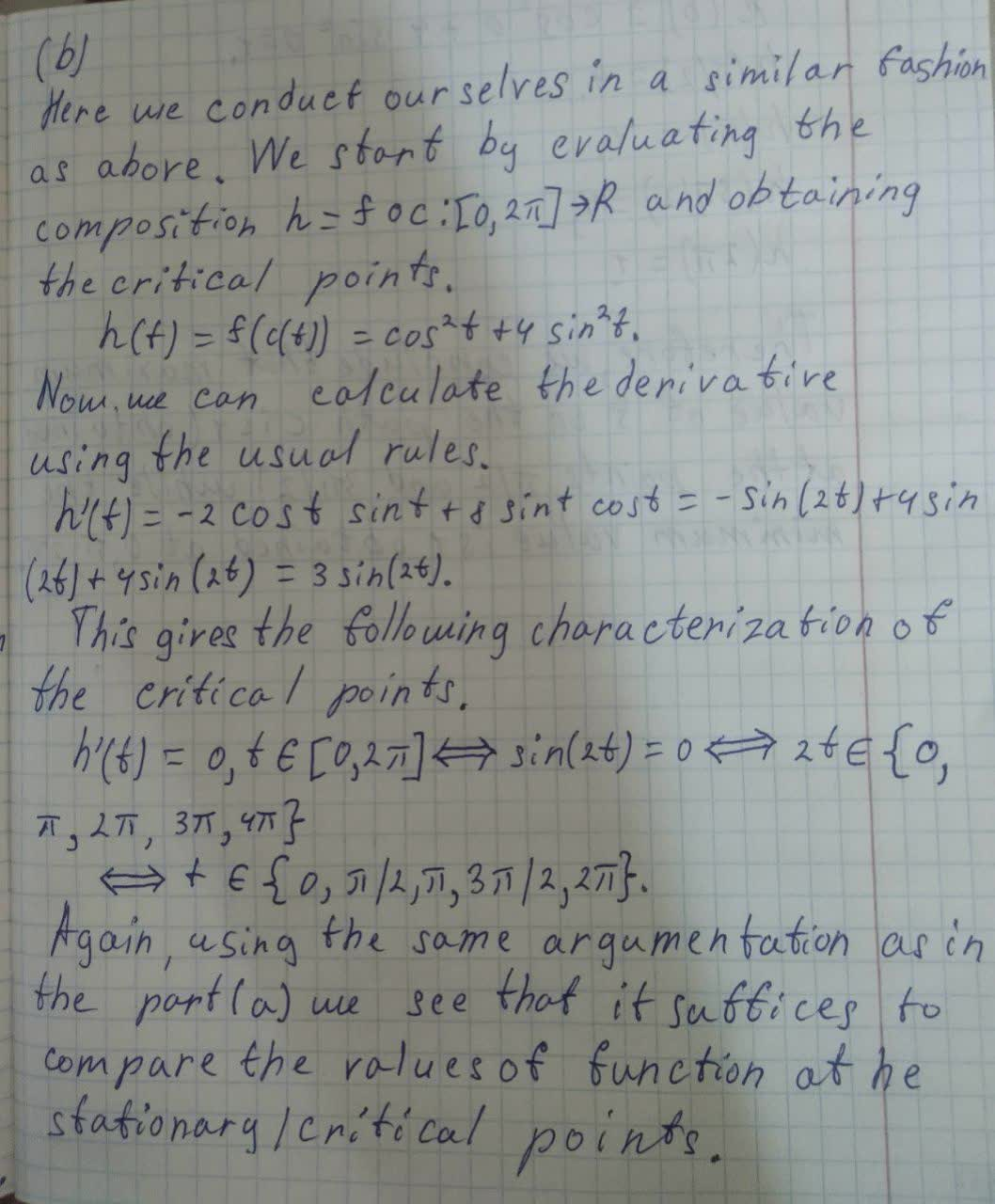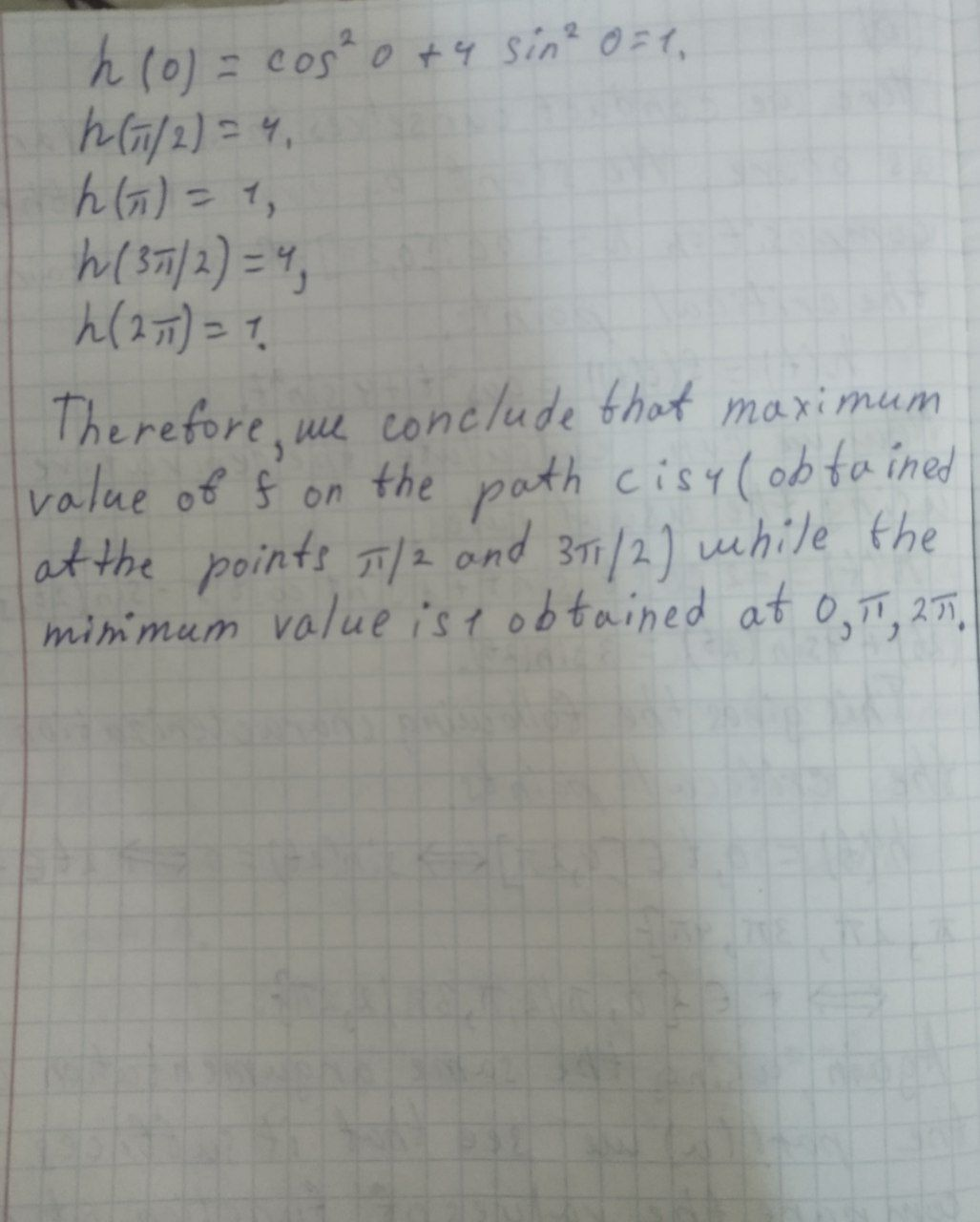# For each of the following, find the maximum and minimum values attained by the function f along the path c(t): (b) f(x,y) = x^2 + y^2. c(t) = (cos t, 2 sin t).0 \leq t \leq 2 \pijernplate8 2021-08-03 Answered
For each of the following, find the maximum and minimum values attained by the function f along the path c(t):
(b) $$\displaystyle{f{{\left({x},{y}\right)}}}={x}^{{2}}+{y}^{{2}}.{c}{\left({t}\right)}={\left({\cos{{t}}},{2}{\sin{{t}}}\right)}{.0}\leq{t}\leq{2}\pi$$

• Questions are typically answered in as fast as 30 minutes

### Plainmath recommends

• Get a detailed answer even on the hardest topics.
• Ask an expert for a step-by-step guidance to learn to do it yourself.funblogC